C Programming

# C2000 Solar MPPT Tutorial Pt/4

In this fourth and final part of the C2000 Solar MPPT Tutorial, the system set-up and testing will be looked at.  This will involve the overall hardware set-up for testing, ADC calibration, reduction of noise on the ADC sampling, efficiency test and some improvements for future iterations.  The final C2000 solar MPPT tutorial C code is also downloadable at the bottom of the page.

## System Testing

Once the hardware was calculated and designed, the PCB was laid out and then printed using the freeware version of EagleCad.  The PCB was then printed on a LPKF milling machine, the finished component side of the PCB can be seen in the image below.The board was given a thorough visual check, it was noted some of the drill holes on the component side were marginally out of alignment.  This is due to a slight misaligned of the milling machine alignment pins, the bottom of the PCB is milled first and then flipped so the component side can be milled.  When the board is flipped the minor misaligned becomes apparent, it is not enough to cause an issue, but I manually make all my own footprints allowing for the small idiosyncrasies and tolerances of the milling machine.

The components used for the design were mainly sourced from the education institution stock, with only the INA138 being ordered in.  This reduced any unwanted lead times and also keeps costs down.  There are down sides to this which were found with the IRFI640G MOSFETs.  The mistake was made of soldering these directly onto the PCB without checking the MOSFETs first, they were sourced from a large bag of perhaps 500 or more IRFI640G, all previously reclaimed from other boards.  Once the board was constructed it was noted the power supply was going straight into over current protection, this was narrowed down to two faulty MOSFETs that were short circuit, between Drain and Source.  A quick on the fly test I used to check the MOSFETs can be seen in the image below.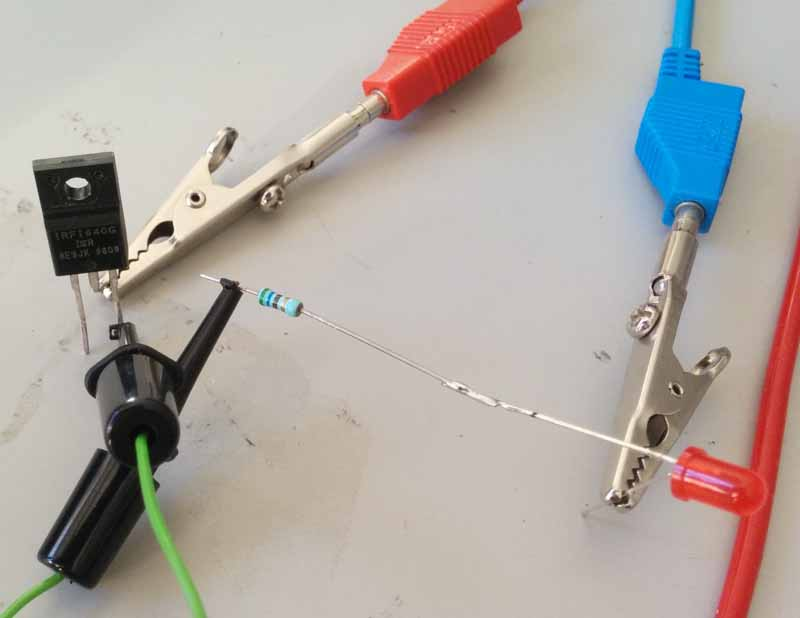## System Calibration

Once the board was working, the next step was to test the buck circuits under controlled conditions,  The test involved the C2000 being set-up to supply a fixed PWM output of 50% duty cycle on PWM1, with PWM2 180o out of phase.  A dummy load in the shape of a 100W potentiometer set to 50Ω was connected to the output terminal, with a 20V supply applied to the solar panel input pins.  A multimeter was used to then measure the output DC voltage, which was observed to be 10V, this confirmed the buck circuit was operating correctly, the 20V was adjusted down to approximately 15V and the voltage was observed to half on the output.

After this initial test the ADC values being sampled needed to be calibrated, this would involve measuring the input voltage and current as well as the output voltage and current.  To achieve this to a good accuracy the same test conditions were used and two Hewlett Packard 34401A multimeter’s.  Some constant values were calculated from the signal conditioning circuitry (see the second part of this tutorial here) used to sample the ADC values, these were calculated as follows.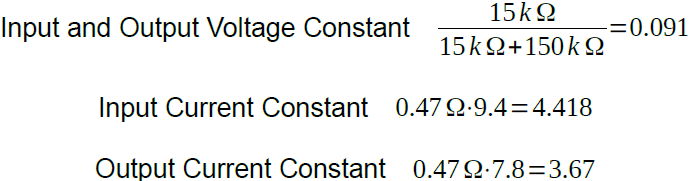These values would then need to be adjusted to meet the tolerances of the circuit.  A laptop was also set-up running Code Composer Studio (CCS) and the calculated ADC variables were viewed, and then the constant calculations values altered until the accuracy was satisfactory. The actual ADC constant values used can be seen in the below code snippet

Various test were carried out at this point to see how the ADC calibration and sampling was working, experimenting with sample and hold times, over sampling.  A useful tool is the graphing feature in CCS this allows trends to be observed in variables.  The original set-up used a timer to trigger the ADC sampling, however this generated more noise due to the MOSFET switching, so the PWM was used to trigger the ADC SOC.

The next image shows a screen capture from CCS, with the debug mode in operation.  The MPPT circuit was supplied with 17.5V from a regulated power supply, the load potentiometer was set to 40Ω, a PWM frequency of 25kHz (not 15kHz) with a 50% duty cycle was used in an open loop configuration.What this graphed data shows is a 2 minute sample window (click the image to expand), the top graph is the input current with a variation of 2.4mA, and the bottom graph input volts with a variation of 10mV.  At the top right of the graph the ADC input variable values can also be seen, the two highlighted in yellow just indicates the value has just changed.

## Efficiency Test

This basic efficiency calculation test was made by graphing the the input and output power under different power conditions.  The power efficiency fluctuated between 85~93%, with the greatest efficiency being achieved towards mid-range power.  The graphed data can be seen below with the input power at the top and the output power at the bottom.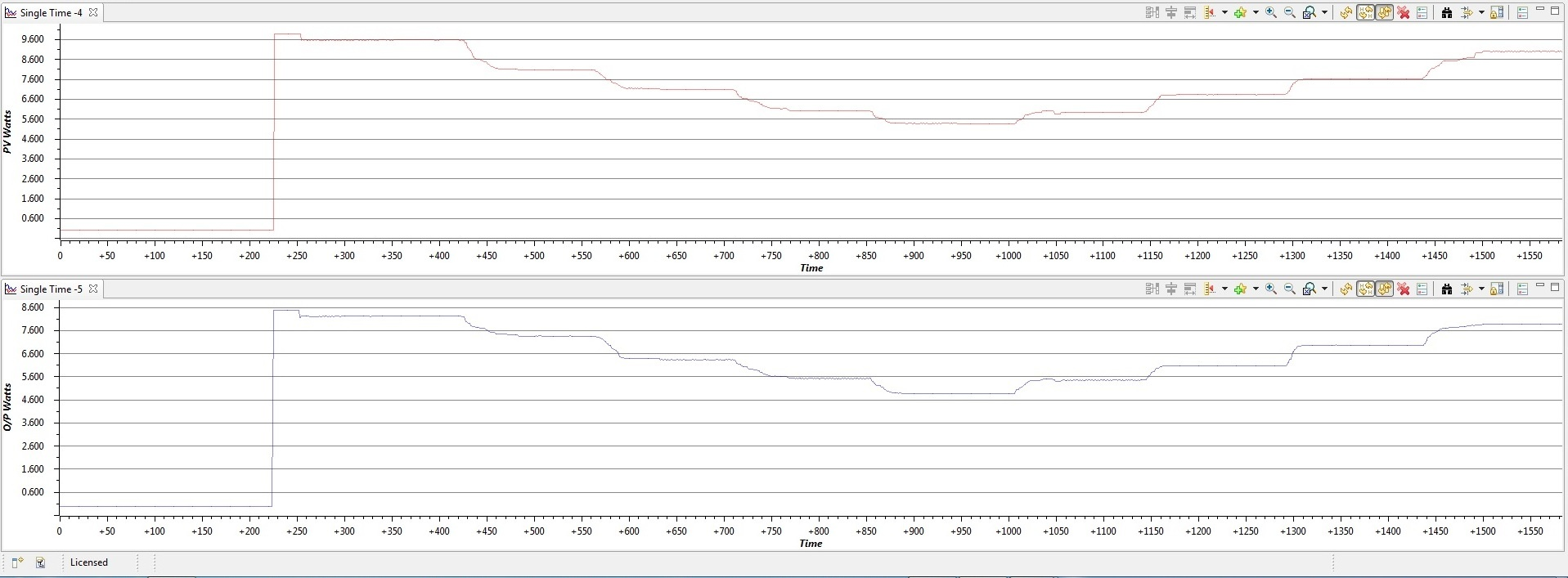## Improvements

This was a prototype design and the first attempt at a solar MPPT, as such there are some improvements that can be made for future iterations.

On the software side, the clock speed for the C2000 could be reduced as there is plenty of idle time, the processor could also be placed into a low power mode between timer interrupts, this would bring an overall reduction in power dissipation.  Additionally a battery charging statement machine could be easily added, with a further form of regulation to switch between full charge and float charge.  If the circuit did not require an interleaved design, the code structure and algorithm could be easily ported across to a lower power and less expensive microcontroller like an MSP430G series.

On the hardware, the MOSFETs are key components that could be changed making the system more efficient.  Greater use of surface mount devices would reduce the circuit trace lengths and noise.  An improved ground plan design would also help reduce noise.  The trace lengths for each circuit phase ideally need to be identical, this will help to balance the phases.  It is possible to sample the current in each phase, then use software to adjust the duty cycle to correct any imbalances, however this increases the overall systems complexity particularly the software.

## C2000 Solar MPPT Tutorial C Code Download

The link below contains the zip file with the complete C code, there is a small advert page first via Adfly, which can be skipped and just takes a few seconds, but helps me to pay towards the hosting of the website.

C2000 Solar MPPT Tutorial Full C Code

I take great care when writing all the tutorials and articles, ensuring all the code is fully tested to avoid issues for my readers.  All this takes time and a great deal of work, so please support the site by using the Adfly links etc.  If you have found this useful or have any problems implementing, please feel free to leave a comment and I will do my best to help.

# C2000 Solar MPPT tutorial Pt/3

In this third part of the C2000 Solar MPPT Tutorial, the software will be looked at in greater detail.  This will entail a look at the Perturb & Observe algorithm, ADC code and timing code to ensure everything operates in a controlled manner.

The software is based around a Perturb and Observe (P&O) algorithm, the P&O algorithm falls under the category of a hill climbing algorithm.  Hill climbing algorithms are named so due to the algorithm taking steps over sampled data to reach a desired value, in the case of the P&O this takes steps towards the MPP by increasing or decreasing the duty cycle.  There are other hill climbing algorithms such as dP/dV Feedback Control and Incremental Conductance, I intend to revisit these and write code at a later date, but for now will focus on the P&O.  Some further information on MPPT algorithms can also be found here.

## Perturb & Observe Algorithm

The P&O algorithm is a relatively simple algorithm, as such it has a few drawbacks:

• The algorithm can be confused and track in the wrong direction, this can occur under fast changing irradiance conditions, the severity of this confusion depends on the P&O setup i.e. step size and update frequency.
• The algorithm oscillates around the set point showing characteristics of an on/off controller.  More on this can be found on a previous tutorial I wrote regarding PID control, which can be found here.

The flowchart below shows the P&O algorithm used for this projectAs can be seen from the flowchart the algorithm is fairly easy to follow, turning this into C code is also relatively easy, the final C code P&O algorithm can be seen below inside the function Adj_PWM().

So lets now run through the code briefly starting with line 3, this basically assigns the value in the counter compare A register to variable PWM_Temp.  PWM_Temp could simply be assigned to a temporary global variable, but I chose to get it straight from the register in this case.  Lines 5 to 24 form the main body of the algorithm, looking back at the flowchart the first two steps “Sample” and “Calculate” are carried out elsewhere in the ADC section of the code.  Lines 5 to 14 are illustrated by the right hand branch of the flowchart and lines 15 to 24 are illustrated by the left hand branch of the flowchart.

You have some simple if and else statements that determine which direction the algorithm takes, which is dependant on the sampled ADC data.  The result of these steps will either increase or decrease the PWM duty cycle, this increase or decrease determines the step size and in this case that value is 2.

The next block of code from lines 26 to 31 are used to prevent the duty cycle from reaching too large, and too small a value.  This was used during tuning, but also serves to provide some boundaries for the PWM, for example the duty cycle for the half bridge MOSFET drivers cannot exceed 99%, or the boost function will not operate correctly.

Lines 32 and 33 are used to update the duty cycle to the counter compare A registers for PWM1 and PWM2, both are the same duty cycle but PWM2 is 180o out of phase with PWM1.  Line 35 then assign the latest calculated solar panel voltage IP_Volt to the variable Old_IP_Volt and line 36 assign the latest calculated solar panel power New_PW_In to the variable Old_PW_In, both these variables are then used when the Adj_PWM() function is called again.

In order to help visualise the two PWM signals, the below image shows an oscilloscope trace with PWM1 in yellow and PWM2 in blue, both are set to 50% duty cycle and PWM2 is out of phase by 180o with PWM1.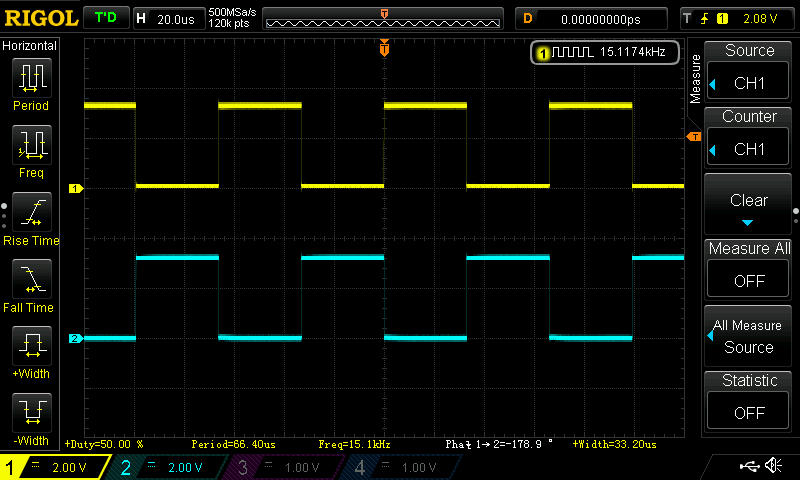The next piece of code to be looked at is the ADC, I am not going to show the set-up code for the ADC or the PWM that triggers the ADC SOC, but will just show the code relating to the sampling and calculation.  However I intend to write a tutorial on each peripheral inside the C2000 with code examples, when time allows.  The ADC sampling is triggered by the PWM on every first event, therefore the sampling rate is 15ksps.  The final ADC sampling code can be seen below inside the function Data_Update()

So starting with lines 3 to 6 these are the local variables used for the function, the two floats are used to store the ADC values and the two integers are used to determine how many samples in the for loop.  The float in line 4 is a float array with four arrays, now the same result could be achieved with four separate floats.  I have left it as a float array for now, but if four floats were used the code should be optimised, by using 64 samples the following division of 128 (lines 23 to 26) could be substituted with a right bit wise shift of 7.

Lines 23 to 26 divide the sum_of_ADC_samples_Array[n] by 128 and assign the value to ADC_An floats.  Lines 28 to 31 convert the new floats to real world voltages read on the GPIO. Lines 33 to 36 then use constant values calculated from the electronic component values in the circuitry, to convert the floats to actual voltages and currents sampled in the circuit.  Lines 38 to 40 simply convert the input voltage and current to an input power and the output voltage and current to an output power.

## Timing Code

The timing code is quite critical as it determines the update frequency of the MPPT, it must also ensure the code does not overrun and cause unpredictable behaviour.  The internal timer module was used, Timer 0 was set-up to trigger an interrupt every 100mS or 10Hz. The interrupt code is shown below.

When the interrupt is called an integer called SysTick is set to one, then the interrupt flag is cleared allowing the interrupt request to be executed and exited quickly.

Inside the main function there is a continuous while loop, the following code is run inside this loop.

Every time the interrupt sets the integer called SysTick to one, it allows the functions Data_Update() and Adj_PWM() to be executed, once these functions have completed SysTick is set to zero.  There are some additional lines of code on line 5 and line 10, these are used for testing and allowing the code execution time to be displayed on an oscilloscope.  The code on line 5 switches GPIO pin 19 high, then the code on line 10 switches GPIO pin 19 back to low, so a square wave pulse is produced and the pulse width gives an indication of the code execution time of the functions Data_Update() and Adj_PWM(). The following images show captures from an oscilloscope.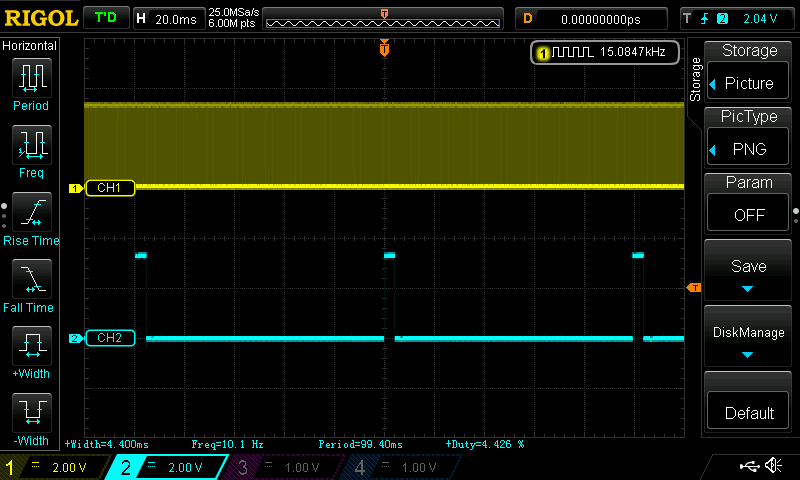This first image shows the 15kHz PWM being displayed on channel 1, the individual wave pulses are not visible as the time base is set to display channel 2.  The blue trace shown on channel 2 can be seen to have a frequency of 10Hz, with a pulse width of 4.4mS, so the functions Data_Update() and Adj_PWM() take 4.4mS to execute.  Putting this into context there should be 128 ADC samples captured, taken from 64 triggers of the PWM signal, therefore 64*66.67uS (one 15kHz cycle) = 4.27mS.  A single ADC sample and conversion takes around 650nS, if we multiply that by the 8 samples, a conversion is being completed every 8*650nS = 5.2uS (it will be faster than this due to ADC pipelining effects).  It can be clearly seen that there is plenty of room for more oversampling if required, as the 5.2uS sample and conversion time easily fits inside the 66.67uS window of the PWM trigger.  There is also a small amount of code overhead being added artificially by toggling GPIO pin 19, which is not significant but something to be aware of.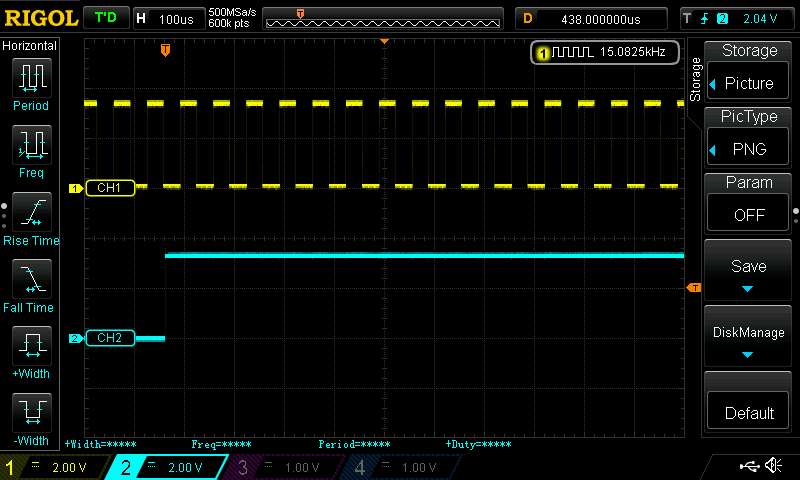The second image has a smaller time base setting (100uS) effectively zooming in, which allows the individual pulses from the 15kHz PWM to be visible.  So going back to the step size of 2 shown in the Adj_PWM() function, this can be put into context when the maximum duty cycle value as a variable, for 100% duty cycle equals 1000.  Therefore with a PWM update frequency of 10Hz and a maximum step size of 2, this equates to a maximum duty cycle change of 2% per second.

I captured some video which shows 3 variables being graphed in Code Composer Studio, these variables are PV Power, PV Volts and the Duty Cycle.  The video also demonstrates the MPPT in action under simulated fast changing cloud conditions, as well as some natural cloud.

There will be one final part to this series of tutorials this will cover some of the set-up and testing, and will also include a link to the full C code for the project.

I take great care when writing all the tutorials and articles, ensuring all the code is fully tested to avoid issues for my readers.  All this takes time and a great deal of work, so please support the site by using the Adfly links etc.  If you have found this useful or have any problems implementing, please feel free to leave a comment and I will do my best to help.

# PID Tutorial C code Example Pt/2

In this PID tutorial C code example Pt/2, the basic concept of a Boost PID controller will be explored.  All the PID controller C code is stepped through, as well as some basic concepts for testing and tuning the controller.  The final C code example can be downloaded via the link at the end of the article.

## Hardware Overview

The image below shows a rough blocked out diagram of the boost circuit and feedback loop.As can be seen it’s a fairly simple design, with the output voltage being monitored and fed back to the LM3S6965.

A schematic for the boost converter can be seen below, this circuit was originally made to test a variable voltage boost converter.  The original design would use a 5V input which would then be boosted to either 9V, 12V or 15V, this was controlled via a state machine running on the Stellaris LM3S6965.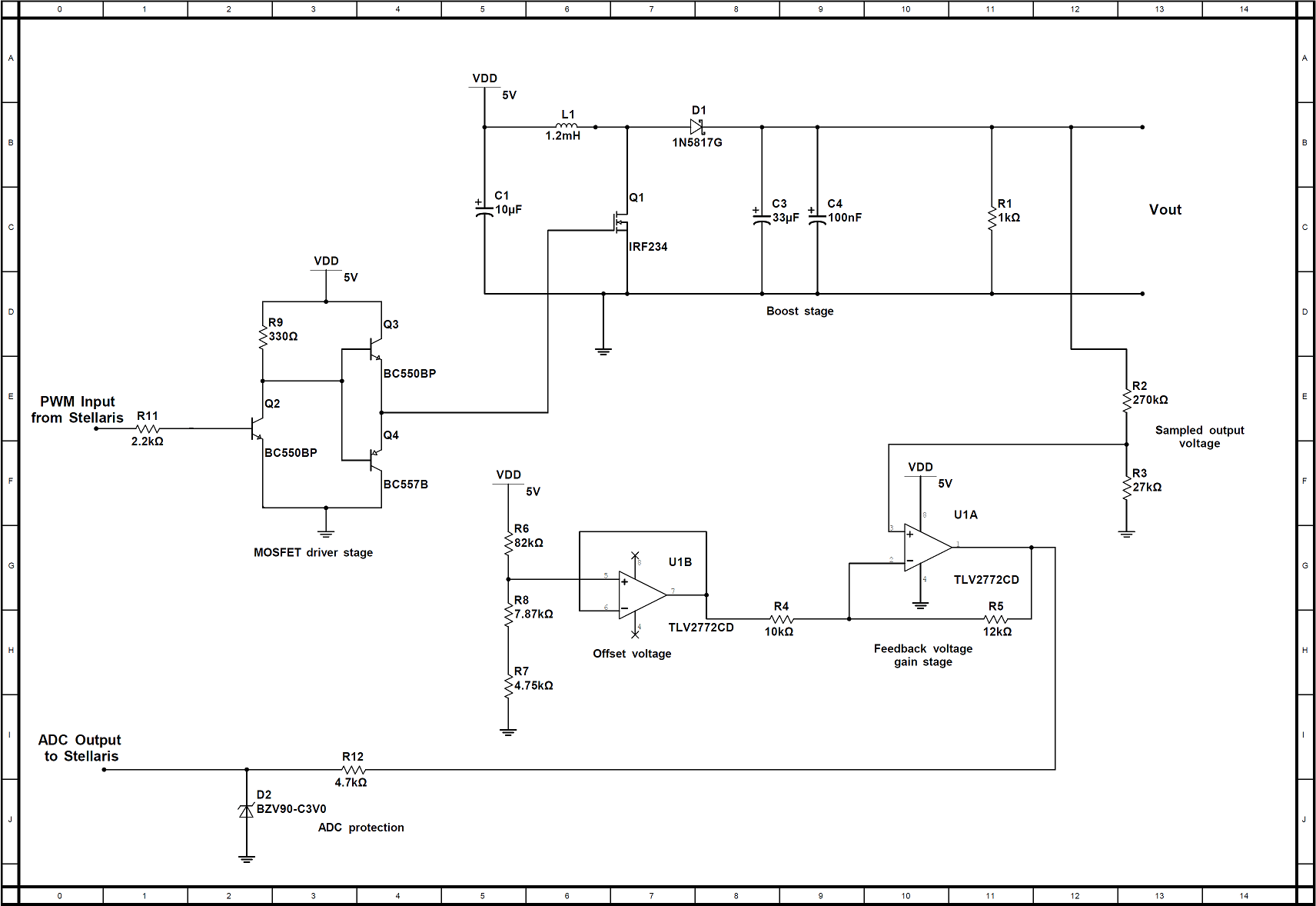The drive circuit for the mosfet uses 2 transistors in a totem pole configuration, and a 3rd transistor is to ensure a small current is sourced from the LM3S6965.  The main boost circuit itself consists of L1, Q1, D1 and C3.  The sampled output voltage via the potential divider is first passed through an operational amplifier U1A, configured as a non inverting amplifier which provides a gain of 2.2.  The operational amplifier U1B acts as a unity gain buffer, it’s input is connected to 3 resistors R7, R8 and R9.  These 3 resistors provide the offset voltage of 770mV and U1B ensures there is a high impedance input.  The output of U1B feeds the offset voltage to R4 and then to the negative input on U1A (effectively offsetting ground).  The boost converter was originally set-up to measure voltages between 4V and 19V.  So the operational amplifier arrangement with offset, ensures the sampled voltage range uses almost the full range of the ADC.  For the PID testing the output voltage will be regulated to 9V, which is equal to 353 on the ADC which is also the set point value.

## Software

The software used was written after reading various sources on PID.  It has been designed so it is easy to understand, as it uses local and global variables.  A more efficient way would be to use a typedef structure or typedef struct in C.

So firstly we have our constants and global variables.

In the first part of this tutorial the proportional (Kp), integral (Ki) and derivative (Kd) constant values were mentioned.  These are the values which provide the tuning for the PID control system.  The next constant is the set point, this was observed as the read ADC value, when 9V was present on the output.  The 9V was measured and adjusted using a multimeter with a fixed load on the output, the duty cycle had a fixed value which was then increased until 9 volts was reached.  The first 2 global variables are used to store the accumulated and historic error values required for integration and differentiation.  The global variable PWM_Temp is initially given a value, then this is used to store the old duty cycle value, which is then used in the new duty cycle calculation.

The next set of variables are all local to the PID function called PWM_Duty_Change(), all the code that follows will be inside this function, until the closing brace of the function is stated and mentioned.  Additionally the entire code which was used on the LM3S6965 can be downloaded (bottom of the page), so it can be seen how and when the function was called.

The first 2 local variables are called iMax and iMin, these are used to prevent integral windup.  More will explained on integral windup further in the tutorial, but for now we will just run through the variables.  The next 4 variables have already briefly been touched upon in part 1 of this tutorial, these are Err_Value, P_Term, I_Term and D_Term.  Then we are left with new_ADC_value which is simply the latest sampled value form the ADC, and finally PWM_Duty which will be the new PWM duty cycle value passed to the timer module.

The read ADC code does not need much explanation, other than a function was created to read the ADC and return the value as an integer.  If you are using this code on another microcontroller, you simply need to construct an ADC read function, then insert instead of the read_ADC().

Going back to part 1 of this tutorial it was shown that the error value can be obtained, by simply taking the newest error value away from the set point, that’s all this line performs.

To obtain the proportional term the Kp constant is multiplied by the error value.

The accumulated error value is used in integration to calculate the average error, so first the current error value must be added to the accumulated value, and that’s what this line performs.

The if and else if statements are used to cap the accumulated integral term, by simply setting upper and lower thresholds.

The integral term is then calculated by multiplying the Ki constant with the accumulated integral value.

The first line of this code snippet calculates the derivative term.  To understand how the code works the second line needs to be run through quickly, this basically assigns the newest ADC value to the d_temp global variable.  So the d_Temp variable effectively stores the historic error value, therefore allowing the derivative to be calculated.  Now looking at the first line again, the newest ADC value is taken away from the historic value, the difference between the 2 would allow the rate of change to be calculated (assuming the time between the readings is a known quantity). Then this value is multiplied by the derivative constant and assigned to the derivative term.

Now that we have all the PID terms calculated, they can be used to change the PWM duty cycle.  This next piece of code adds all the PID terms together, then they are subtracted from the PWM_Temp variable (PWM_Temp usage is shown shortly as it comes towards the end of the function).  This value is then assigned to the PWM_Duty variable.  The way the PID terms are used here is specific to this program, firstly the sum of the PID terms is subtracted, this is due to the final electronic circuit used.  What’s important here is the PID terms are summed, then they need to be used in such away as to construct a valid PWM duty cycle or other chosen control method.

The if and else if statements are used to prevent the duty cycle from going too high or too low, in this case this simply prevents the output voltage from going too high or too low.  If say you were using a half bridge driver integrated circuit, these usually state a maximum duty cycle of say 99%, if this is exceeded the voltage doubler cannot function correctly, so having limits can be a good protection system.

This line is again limited to this specific application, it simply calls the function adjust_PWM(), which has the new PID calculated duty cycle value, as a parameter.

The final statement in the function before the closing brace, assigns the current PWM duty cycle value to the PWM_Temp global variable.

Integral windup can occur if the integral term accumulates a significant error, during the rise time to the set point.  This would cause the output to overshoot the set point, with potentially disastrous results, it can also cause additional lag in the system.  The integral windup prevention used in this example, places a cap on the maximum and minimum value the integrated error can be.  This effectively sets a safe working value and is considered good working practice in any PID system.  The values used for integral windup as with the PID constant gain values are specific to this system, these would need to be determined and tuned to your system.

## Step Response

The intention was to also explore the well documented Ziegler-Nichols approach, however time constraints for the project did not allow this method to be fully explored.  Some basic concepts of how to carry out a step response test will be shown, as I am sure it will prove useful.

The systems step response shows how the system responds to a sudden change, electronic circuits switch quickly, so an oscilloscope was the best piece of equipment for the job.  The boost converter was set up with a fixed load, a fixed PWM duty cycle and no feedback , therefore in an open loop configuration.  Then some additional test code was added, initiating these 2 extra functions when a button was pressed on the LM3S6965 development board :

1. A free GPIO pin would be taken from low to high
2. The PWM duty cycle would decrease by 10% effectively increasing the output voltage

The free GPIO pin would be connected to channel 2 on the oscilloscope, and in this way can be used as a trigger to start a capture event.  Channel 1 on the oscilloscope would be connected to the output of the boost converter, thereby capturing the step response as the output voltage rises.  The image below is a photograph of the captured step response on the oscilloscope.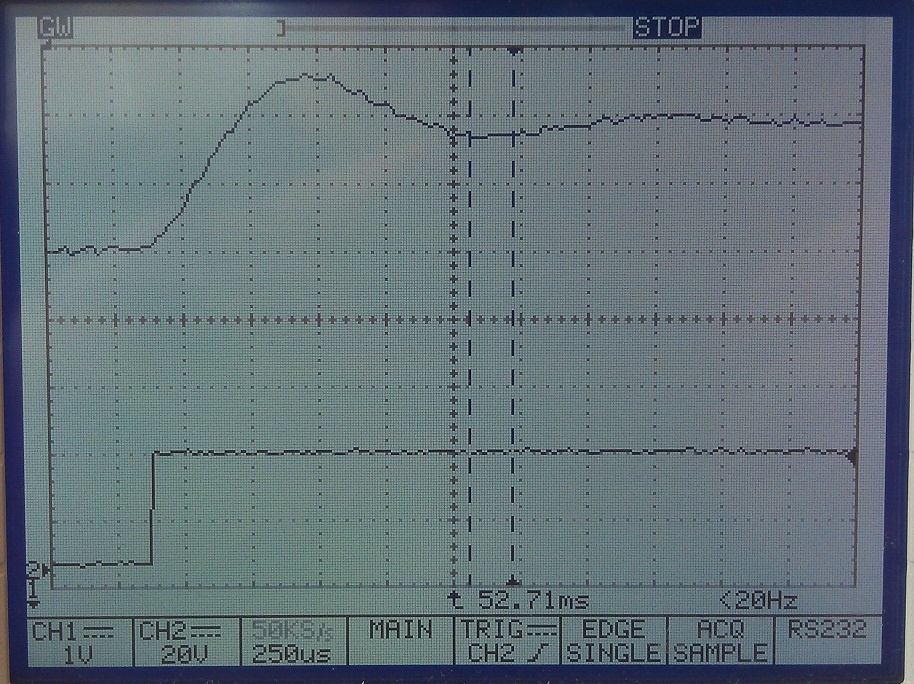The GPIO pin was polled before the PWM duty cycle was updated, however this happens so quickly, it is difficult to distinguish this.  The lower square wave type step is the GPIO pin on channel 2, and the top curved step response is the output from the boost converter.  It can be seen to overshoot it’s settling level at the beginning then oscillate for a time before settling down, this oscilloscopes accuracy wasn’t the best, using a longer time base did not show any extra detail on the oscillations.

If the step response was of a first order, a calculation based on the reaction curve could be performed.  However the step response from the boost circuit is of a second order, and has an under-damped nature.  In order to explore this calculation further, requires another tutorial altogether, however further reading on this can be found here.

The step response of a similar system under the control of a PID controller can also be viewed.  This can be achieved by using a similar set up, the external pin is used again to trigger an oscilloscope capture.  That same GPIO pin is also used to switch a mosfet, which places an additional load in parallel with the output of the boost converter.  The PID algorithm will then regulate the output voltage to ensure it meets the set point with the new load, and the step response can then be captured.

## Testing and Tuning

The system was set up and a basic tuning approach was used, the image below shows the system on the test bench.The image shows the following pieces of equipment:

• Hewlett Packard multimeter connected to the output, monitoring the output voltage
• Bench power supply, supplying 5V to the circuit
• Oscilloscope, used to monitor the PWM duty cycle
• 0-100Ω bench potentiometer
• Boost converter PCB, LM3S6965 development board and a laptop to tweak the code

With everything connected the PID algorithm was set up, but with only the proportional part in operation.  The set point was set to 353 as mentioned previously, which produced close to 9V give or take 10-15mV.  The load was fixed and set to 100Ω.  Initially the proportional gain constant Kp was set very low around 0.001, this was too low so it was increased over a few steps until 0.01.  At this point the output voltage was observed to be approximately 7.5V, and the duty cycle waveform was observed to have a small amount of jitter, as the duty cycle fluctuated +/- 0.5%.  The Kp value was again increased, until the output voltage read approximately 8V, the duty cycle was again observed and found to be fluctuating by a greater amount, by approximately +/-5%.  The increase in jitter on the PWM duty cycle can be equated to greater oscillations on the output voltage, the multimeter was not suitable for observing these.  It would have been possible to see them on the oscilloscope but this probably would have involved a stop capture then restart method, therefore to make the process quicker the PWM duty cycle was considered ‘good enough’.

At this point the Kp value was backed off, to the previous level of 0.01 so the output voltage was at 7.5V.  This point can be considered close to the offset level mentioned in part 1 of this tutorial, and for ease of reading the image showing the offset level can again be seen below. This image also illustrates again how too larger Kp value can introduce instability in the output.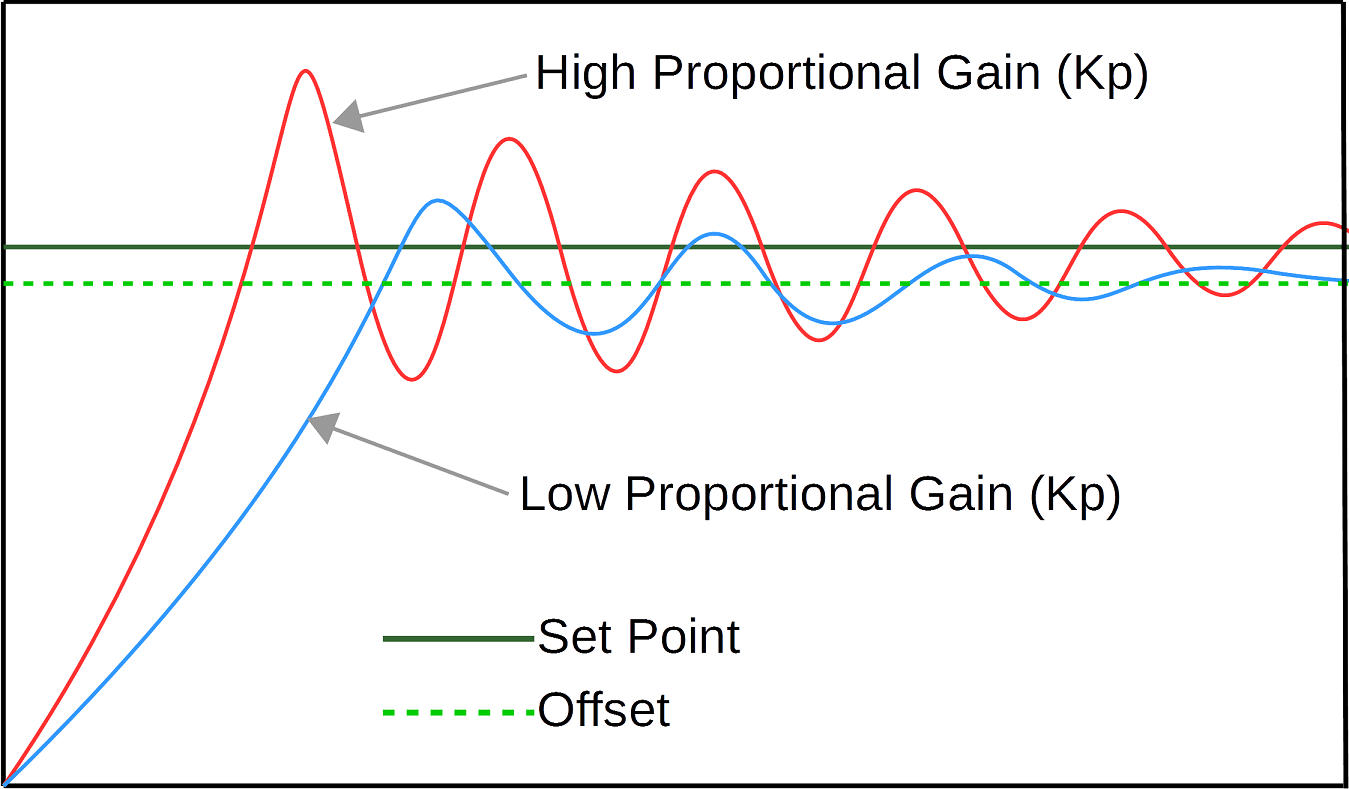Now with this level reached, the integral part of the algorithm was introduced.  All the hardware was untouched, just a simple modification to the algorithm was made.  The initial value chosen for Ki was 0.01.  This was then loaded on to the LM3S6965 and the output voltage on the multimeter was immediately observed to read 9.02V, +/-10mV.  The oscilloscope was also observed to have minor jitter as before of only a few half percent.  The integral value was not tweaked any further, but in most cases tuning the Kp and Ki values would take a greater time and under a wide range of conditions.

With the PI controller working on a basic level, the next test was to see how it performed under different load conditions.  First the bench potentiometer was left at 100Ω and the input current and output voltage were noted, the wiper was then moved to the middle (approximately 50Ω) and again the output voltage and input current were noted.  At 100Ω the output voltage was 9.02V with a current of 180mA, at 50Ω the output voltage was 8.99V with a current of 500mA, both output voltages still showed minor voltage variations in the +/-10mV range.

The next step was to move the potentiometer wiper rapidly between the 100Ω and 50Ω position, then observe the output voltage and duty cycle (not the most scientific test, but goes some way to demonstrating the system).  With this test being performed the duty cycle was observed to rapidly increase and decrease, too quick to observe anything further and a step response would be the best method to use here.  The output voltage was observed to have a minor variations, fluctuating +/-15-20mV

After this test, the derivative constant was introduced and tweaked to find the best settings. The Kd constant was not observed to have a huge affect on the system, unless increased dramatically which produced instability.  This set up is not ideal for testing the derivative term and a more detailed and longer term approach would be best.

The basic test performed seemed to conclude the PI controller was working as per the research carried out.  The system used here updates at a very fast rate, therefore observing the step response at this point would be best practice.  As this system has no critical importance other than to explore PID, it simply wasn’t necessary to tune it to a high degree of accuracy.  I have a number of projects in the works however, which touch on this subject again and I intend to post them when I have time.

## Example Code

The link below contains the zip file with the complete C code and an external functions folder, there is a small advert page first via Adfly, which can be skipped and just takes a few seconds, but helps me to pay towards the hosting of the website.

PID LM3S6965 C code

I take great care when writing all the tutorials and articles, ensuring all the code is fully tested to avoid issues for my readers.  All this takes time and a great deal of work, so please support the site by using the Adfly links etc.  If you have found this useful or have any problems implementing, please feel free to leave a comment and I will do my best to help.

# PID Tutorial C code Example Pt/1

In this PID tutorial C code example Pt/1, the basic concept of a PID controller will be explored. The full example code is implemented on a Stellaris LM3S6965 microcontroller, and is used to control a simple boost converter circuit, regulating the voltage with a change in load resistance. The boost circuit is not covered in-depth in this tutorial, but the fundamentals of a boost circuit are covered.

In this first part the basic concepts of a control system will be explored, as well as the PID controller.  The second parts covers the code implementation in the C language on the Stellaris LM3S6965, all the code is stepped through and can be downloaded at the end of the second part.

## Basic Control System Concepts

To understand how PID controllers are used, it is important to first understand some basic control system principles.  The image below illustrates the 2 basic types of control system.The open loop system can clearly be seen to have no feedback, therefore if the load changes on the motor, the motor speed will change.  The control unit cannot command the driver to increase or decrease the power to the motor, as it has no knowledge of the speed change induced in the motor, by the change in load.

The closed loop system however has feedback from the motor.  So if the motors speed were to decrease due to an increase in load, the control unit could command the driver to increase the power to the motor, keeping a constant speed.  Common direct current motor control is achieved via PWM, and simply increasing or decreasing the duty cycle will increase or decrease the motor speed.

## On/Off Controller

Using the above closed control loop as an example, the basic operation can be broken down. The feedback signal will often go through some signal conditioning circuitry, before being fed to the control unit.  The control unit will have some reference set point or threshold levels, that it is aiming to keep the motor speed at or between.  If a reference set point is used the new feedback signal could be used to generate an error value in the following way

Error_Value = Set_Point – New_Feedback_Value

We will come back to the Error_Value shortly as this is used in the PID controller, but first lets look at a more simplistic approach using the threshold level.

If threshold levels are used, lets say to keep the motor speed between 1200-1300rpm, the feedback signal could be directly used as a comparison.  Therefore if the feedback signal is 1320rpm the control unit needs to decrease the motor speed.  If we now said the control unit was a microcontroller, and the driver was a simple amplifier.  The microcontroller could reduce the duty cycle of the PWM, this in turn would then slow the motor down.  If we consider the motor to have a very quick response to changes in PWM drive, and the load on the motor to alter subtle over time, then there are 2 important factors to consider.  (1) The speed at which the PWM duty cycle is updated by the microcontroller, and (2) how large a change is made to the duty cycle on each update i.e. 1%, 5% etc.  This control system is often known as an on/off controller, the 2 factors can be tuned to suit the individual control system.  An example of on/off controller which is slightly more complex than this example, as it has other parameters is a Perturb and Observe algorithm used in a solar MPPT for photovoltaics.  The image below shows how an on/off controller usually oscillates around the set output level.On/off control systems typically oscillate around the desired output level, as they overshoot and undershoot the desired value.  The threshold level example used has no fixed setpoint so even if the motor speed stayed within these bounds, its speed would still oscillate to some degree.  The size of these oscillations is dependant on the PWM step size and the control loop speed.  If the system is very dynamic and changing at a fast rate, then a compromise between these 2 factors needs to be reached, to ensure good tracking performance.  When the desired reference value is reached, the size of the oscillations will be dependant on the PWM step size.

## PID Controller

PID stands for Proportional, Integral and Derivative.  PID controllers have been used since the 1890s, and were originally developed by Elmer Sperry for an automatic ship steering system.  A PID control system uses 3 gain constants, which are multiplied with the Error_Value.  The proportional value gain constant is referred to as Kp, the integral value gain constant as Ki and the derivative value gain constant as Kd.   The results of these multiplications produces 3 further values, for this tutorial these are referred to as the P_term, I_term and D_term.

As previously mentioned the Error_Value can be obtained from simply taking the New_Feedback_Value away from the Set_Point.  In a PID controller the error value can simply be multiplied with the Kp constant, but for the Ki and Kd constants a different approach is required.  In order to calculate the I_term and D_term historic error values are required.  The I_term is using integration which gives the average error value over time, and the D_term is using differentiation which gives the rate of change of the error value over time.  Once all the PID terms are calculated, these new values are then summed together and used in the final control output.

The P, I and D terms have different affects on the final system performance, and need to be tuned to the individual control system.  The ultimate aim for any control system is to respond as quickly as possible, without excessive overshoot and oscillations around the set point.  The system response ideally needs to reach a settled state as quickly as possible, the settled state is very subjective and dependant on the application.  A block diagram of a PID control system can be seen below.The proportional term (P_term) provides an output value which is proportional to the current error value.  The Kp constant value determines how large the gain response will be to the current error value.  A high proportional gain constant will induce a large change in the output, often overshooting the set point level.  If the gain is too high the system can become unstable and excessive oscillations can occur.  Another affect of a high Kp constant is faster response time, vice versa a low Kp constant will result in a slower response time.  When a low Kp constant is used, there will still be oscillations and some overshoot of the set point depending on the level, however these will not be as aggressive.

A low Kp value will also settle in an offset position slightly below the set point level, this offset position can be used when tuning a PID system.  The image below illustrates the step response waveforms of a system with high and low Kp gain constants, on a hypothetical control system.The integral term (I_term) is used to bring long term precision to the control loop.  It is proportional to both the magnitude and duration of the error.  Using Integration allows for the average error to be calculated, as the sum of the instantaneous error over time provides an accumulated offset.  The accumulated error is then multiplied by the Ki gain constant, allowing the offset which should have been corrected previously, to be added to the controller output.  This then accelerates the controller output response towards the set point, eliminating the offset found when just using a proportional control value.

As with the the Kp gain constant the Ki constant, needs to be tuned.  If the Ki value is too high the system will have a faster response time, but it will also be likely to overshoot the set point, inducing further unwanted oscillations before finally settling.  An ideal Ki value produces a response time that is fast enough for the application, with little or no overshoot and a quick settling time.  The image below illustrates the step response waveforms with a tuned Kp value already set, then a few Ki gain constants are applied on a hypothetical control system.The derivative term (D_term) is the least used of the three terms, most controllers are based on PI control algorithms.  Introducing the Kd term is generally used in specific control systems, these are usually where overshooting the set point can cause dangerous results. Differentiation is used to determine the rate of change, by using the instantaneous error value and the previous error value, so a rate of change can be determined.  The derivative term can be used to predict system behaviour, improving settling time.

## PID Control Loop Tuning

As can be seen the constant gain values Kp, Ki and Kd all affect the control system in different ways, therefore careful tuning of these values is an essential part of an effective PID controller.  Tuning a PID system is a difficult problem, there are only 3 parameters but finding a balance often takes some time depending on the complexity of the system.  Some systems have auto tuning features, but in some situations to have the best performance from a system, it is often recommended to manually tune.

A well known tuning method is called Ziegler-Nichols, developed by John G. Ziegler and Nathaniel B. Nichols.  This relies on the Ki and Kd gain constants first being set to zero.  The the Kp constant is adjusted until it reaches the ultimate gain, which is classified by the output from the control loop oscillating at a constant amplitude.  Then the ultimate gain value and oscillation period are used to calculate the Ki and Kd constants.  More about the Zieglar-Nichols method can be found here.

There are other methods and simply searching on Google will yield many results.

The C code, set-up and tuning of the boost converter will be covered in part 2 of this tutorial.

I take great care when writing all the tutorials and articles, ensuring all the code is fully tested to avoid issues for my readers.  All this takes time and a great deal of work, so please support the site by using the Adfly links etc.  If you have found this useful or have any problems implementing, please feel free to leave a comment and I will do my best to help.

# C Programming Pointers Pt/1

This first tutorial will be a basic introduction to C programming Pointers.  They are an important part of the C language, providing a powerful and flexible method of manipulating data.  When pointers are used with functions, it allows the values of variables to be changed regardless of where they originated.

## Declaring A Pointer

The code snippet below shows how to declare a basic pointer, there are 2 examples showing an int and a char variable.

So the first part of the code int and char in this case, is the type of variable the pointer points to.  The next part which starts with an asterisk (*) is known as the Indirection operator.  The indirection operator tells the compiler P_Name1 is a pointer to an integer and P_Name2 is a pointer to a char.  So the indirection operator tells the compiler which type of variable the pointer name points to, in this case either an integer or a char.  It is important to note that these are pointers and not variables, the compiler knows this due to the indirection operator, and the context in which it has been used.

Pointers can also be declared along with other non pointer variables, an example can be seen below.

## Initializing Pointers

Initializing the pointer basically ensures the pointer actually points to something.  This is carried out by the pointer storing the memory address, of the variable it points to.  The ampersand (&) or Reference operator is used to do this, as it returns the address of the variable   An example below shows how the pointer is initialized.

The ampersand is placed before the variable we want the pointer to point to.  When placed before the name of a variable, the reference operator returns the address of the variable. The next example demonstrates a similar initialization, but the pointer and integer declarations have been added as well.

The image below shows a screen capture from Eclipse.  The variable Result has been initialized with a value of 10 (this has been done to avoid some random value being displayed), The value of P_Name is the memory address location of Result, and *P_Name has the same value as Result.## Basic Example Of Pointer Usage

The next example builds on the previous code, by using similar named variables.  It also demonstrates how the indirection, and reference operators can be used in a basic form.

The result of this basic program is displayed below with a screen capture from Eclipse.So what we can see here, well the variable Result was declared and initialized with a value of 10,  in line 4.  The first line from the image which reads “Direct Access, Result = 10” shows the value of Result printed, by directly accessing the variable value.  The second line which reads “InDirect Access, Result = 10” demonstrates indirect access to the value of Result, by using the pointer P_Name, which was initialized to point to Result in line 10.Line 15 of the program uses the indirection operator, to print the value stored in the address location pointed to by P_Name.

The third line which reads “The address of Result = 2686696” is produced by line 18 of the code.  This uses the reference operator to print the address of Result, this is the actual memory address of variable Result in the computer memory.  The forth line which reads the same as the third line, uses the pointer P_Name which points to the memory address of Result in line 19.

To summarize whats happening here:

*P_Name and Result both refer to the contents of Result

P_Name and &Result both refer to the memory address of Result

## Computer Memory

At this stage it’s important to talk a little about computer memory as well as variable types.

When a variable is declared inside a C program, the compiler sets aside a unique memory location for that variable.  In the last example the pointer P_Name points to the address of the variable Result, and the program demonstrated how the memory location address could be displayed.  By having unique names for variables it ensures the correct memory location is accessed, the memory addresses are generally hidden from you and not usually used.

Different variable types consume different amounts of memory i.e. int, char etc, also the architecture of the system determines variable sizes i.e. 8 bit, 16 bit etc.  The simple program below can be run on your system.

The output from this program can be seen in the image below, this shows the differing amounts of memory each variable type consume.With this knowledge of how different data types consume varying amounts of memory bytes,  how does the pointer handle the address of multi-byte variables?  Quite simply the pointer points to the first (lowest) address byte of the variable, and the variable size is known by the compiler.

## Examples Code

The link below contains the zip file with the complete C code, there is a small advert page first via Adfly, which can be skipped and just takes a few seconds, but helps me to pay towards the hosting of the website.

Pointer examples code

I take great care when writing all the tutorials and articles, ensuring all the code is fully tested to avoid issues for my readers.  All this takes time and a great deal of work, so please support the site by using the Adfly links etc.  If you have found this useful or have any problems implementing, please feel free to leave a comment and I will do my best to help.

# C Programming Functions Pt/1

C programming functions Part 1, will be a basic introduction to functions in the C language.

A function is an independent part of the program, and can perform a specific tasks without interference from other parts of the program.  Functions can be passed parameters and can also return values.

There are 2 types of functions in C, the existing standard library functions i.e. printf, scanf, gets etc, and user defined functions, that are written for specific tasks by the user.

A user function when used in C programming, should first have a function prototype, then a function definition and then there is the function call inside of the main function.  The image below shows a basic program with a user defined function, illustrating a function prototype, function definition and function call.The function prototype provides the compiler with information about the function, that will be defined later in the function definition.  The prototype contains the return type, the function name and any parameters with variable types the function is passed.  The function prototype ends with a semicolon (;).  The function prototype is usually included before the main function.

The function definition is the actual function.  The first line of the function definition is identical to the function prototype i.e. return type, the function name and any parameters. Additionally the function definition contains all the code that the function will execute, i.e. statements etc.  The function definition unlike the function prototype does not end with a semicolon, but uses curly brackets or braces ({ }), these surround the code that forms the function body.  The function definition can be placed before the main function, but it is often good practice to place it after.

The function call is used to execute the function, and is run from within the main function.

## Defining A Function

A function definition in C programming consists of a function header and a function body.

return_Type – A function may return a value, the return type is the data type of the value the function returns i.e. int, char etc.  A function can also not return a value, in this case the return type would simply be the keyword void.

function_name – The function name is the unique name for the function chosen by the programmer.  There are certain rules that have to abide by for the C language.

parameters – When a function is called, a value is passed to the parameters.  A function needs to know what data type the arguments will be, any of C’s data types can be passed to a function.  If the function takes no parameters then the keyword void is used to indicate this.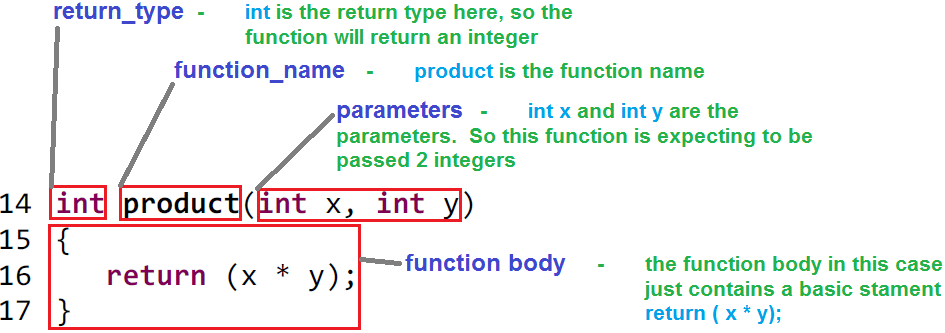## Simple Function Examples

For all these examples Eclipse Kepler was used, there is a small downloadable zip file below with all the full examples including includes etc.

#### Example 1

First lets break down the entire code by line number:  Lines 1 and 2 contain global variables, variables a, b, d and e are all initialized with values.  Line 4 is the function prototype.  The main function starts on line 6 and finishes on line 15.  Line 8 and 9 are both function calls, for the same function but are passed different arguments.  Also line assigns it’s returned value to the variable c, and line assigns it’s return value to the variable f.  Lines 11 and 12 call a C library function called printf, this is used to firstly print the value of the variable c on the screen, return a line and then print the value of f on the screen.  Line 14 is required and in this case the main functions parameter is void, so nothing is returned, hence the zero. Line 17 is the start of the function definition, and also contains the function header.  Line 18 to 20 is the function body and the end of the function definition.

So what does this simple function do?  Well when the program is run line 8 is called first, this executes the product function using variables a and b as arguments.  In the function definition (and prototype) x and y can be looked at as place holders.  So when variables a and b are passed to the function, a is placed where x is and b is placed where y is.  Then the function simply multiplies the 2 arguments together and returns the result.  The result is then assigned to the variable c.

The same is true for the function call in line 9, variables with a different name and value are used, but otherwise it is the same sequence of events.  After this lines 11 and 12 are called and the values of c and f are printed on the screen.  So the result will be c = a*b therefore 50 = 10*5 and f = d*e therefore 25 = 5*5.

#### Example 2

First lets break down the entire code by line number, as with the previous example:  Line 1  contains the first function prototype, this function does not return a value, but accepts 1 parameter.  Line 2 contains the second function prototype, this function returns a value in the form of an integer, but does not accept any parameters.   The main function starts on line 4 and finishes on line 9.  Line 11 to 16 is the function definition for the first function. Line 18 to 24 is the function definition for the second function.

So whats going here?  Well when the main function is called, Line 6 is reached and the first function is called (function_1).  The function_1 is defined as not returning any value, but it accepts 1 parameter in the data type of an integer.  The function_2 is defined as returning a value in the data type of an integer, but not taking any parameters.  So when function_1 is called it then calls function_2, and the return value from function_2 becomes the parameter or argument passed to function_1.  The return value of function_2 is very simple in this case, and just equates to the variable a which has a value of 10.  So the variable a is passed to function_1, using the place holder analogy this value becomes the variable x.  There are 3 local variables inside function_1, these are b (10), c (5) and result.  Then x is multiplied with b and divided by c, the result of the calculation is then assigned to the variable result.  Finally the value of result is printed on the screen using the printf function.

So the final outcome of this program will be resultx*b/c therefore 20 = 10*10/5.

## Examples Code

The link below contains the zip file with the complete C code, there is a small advert page first via Adfly, which can be skipped and just takes a few seconds, but helps me to pay towards the hosting of the website.

Function examples code

I take great care when writing all the tutorials and articles, ensuring all the code is fully tested to avoid issues for my readers.  All this takes time and a great deal of work, so please support the site by using the Adfly links etc.  If you have found this useful or have any problems implementing, please feel free to leave a comment and I will do my best to help.

# Eclipse Doxygen Installation

This is a quick tutorial on how to install Doxygen on a Windows 7 machine, running Doxygen with Eclipse or Code Composer Studio V5.x (CCS).  In the example I will be using CCS v5.4, but essentially this is the same for Eclipse.

Firstly download the latest version of Doxygen from here, at the time of writing this is version 1.8.5. I downloaded the doxygen-1.8.5-setup.exe and ran this to install Doxygen.Once Doxygen is installed open Eclipse or CCS v5.x.  It’s important that the CCS version is 5 or above as older versions do not include the full Eclipse installation, so using all the plugins is not possible.

While CCS is opening a further program is required, which enables the Doxygen frontend to plugin to Eclipse. This program is called Eclox and can be found here .  You need to open this in a browser and scroll down the page looking for the link circled in the below image, then copy this link (will be used shortly).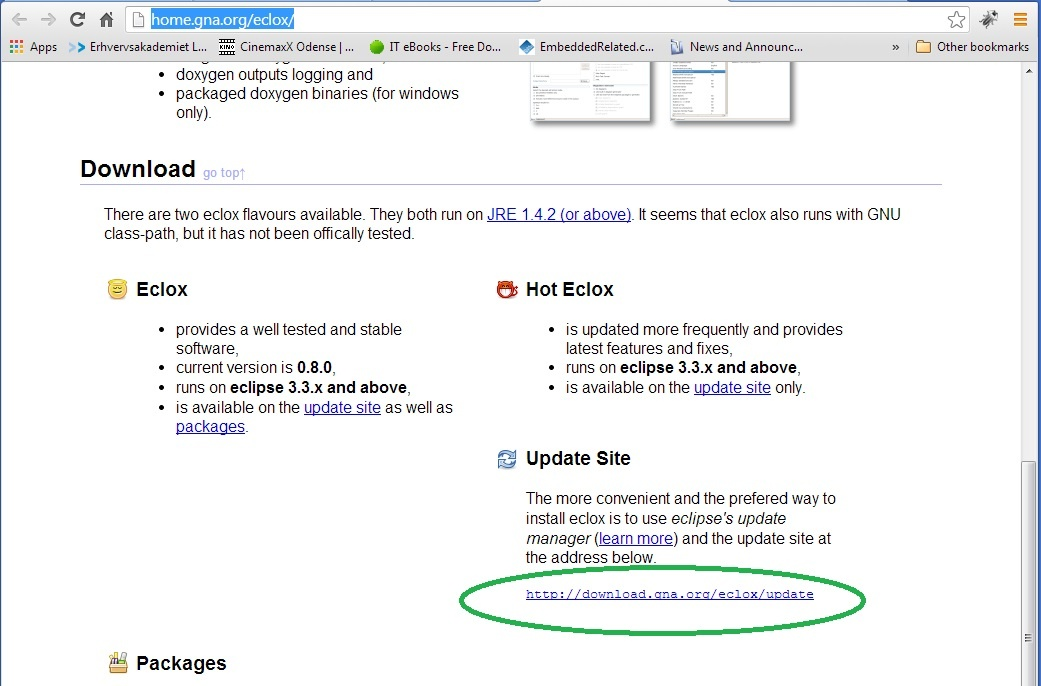With CCS open browse to the Help menu and select Install New Software from the drop down.Once select the Install New Software tab will open up a new window shown below, select the Add button.Then paste the Eclox link into the Location text bar, and click the OK button.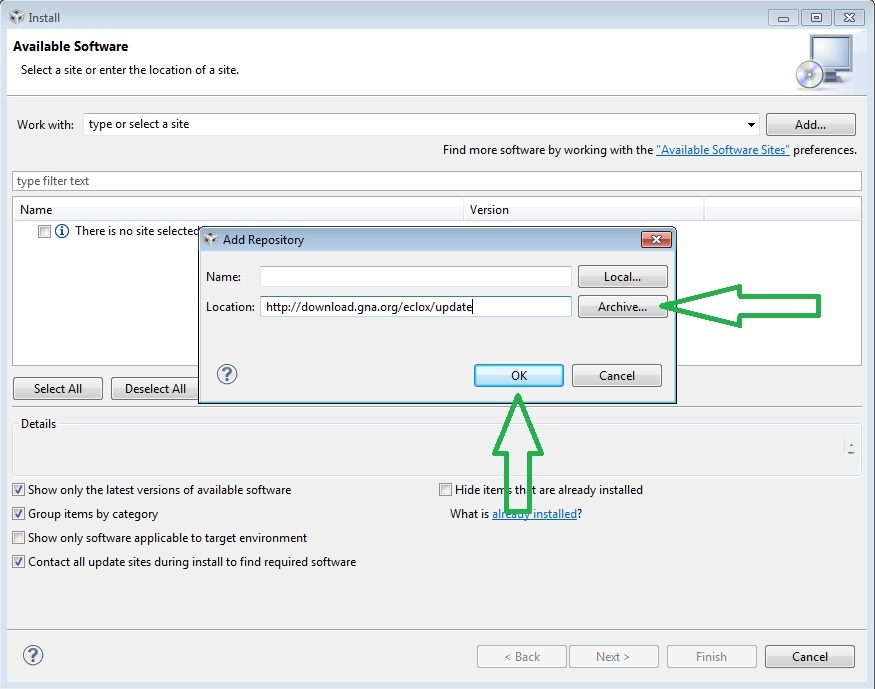This will start a process as CCS checks the link, and when finished you should be presented with a window similar to below.  Ensure the tick box is ticked and press the Next button.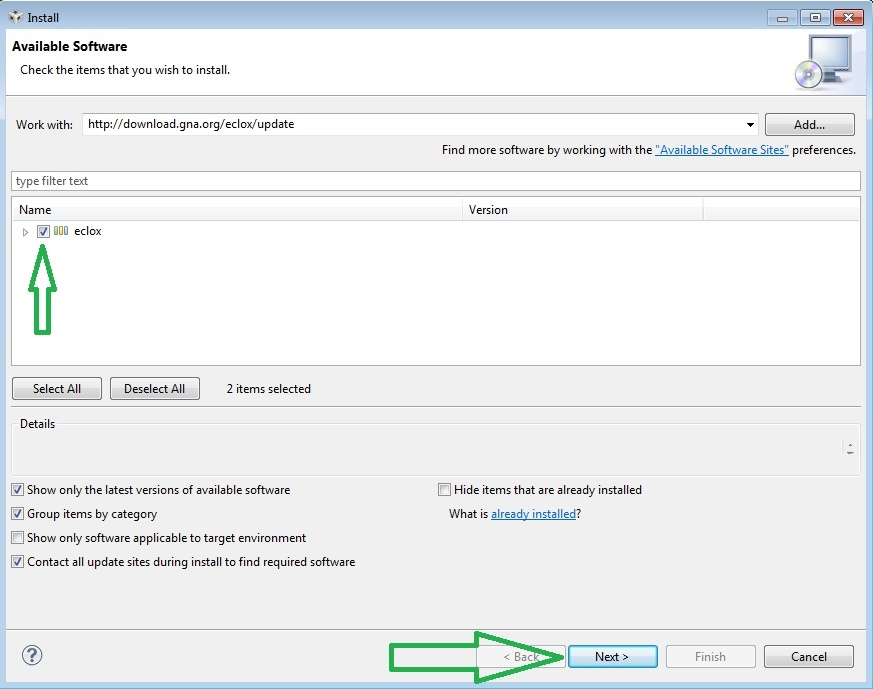The next window is showing the software to be installed, clicking Next will contiune with the installation.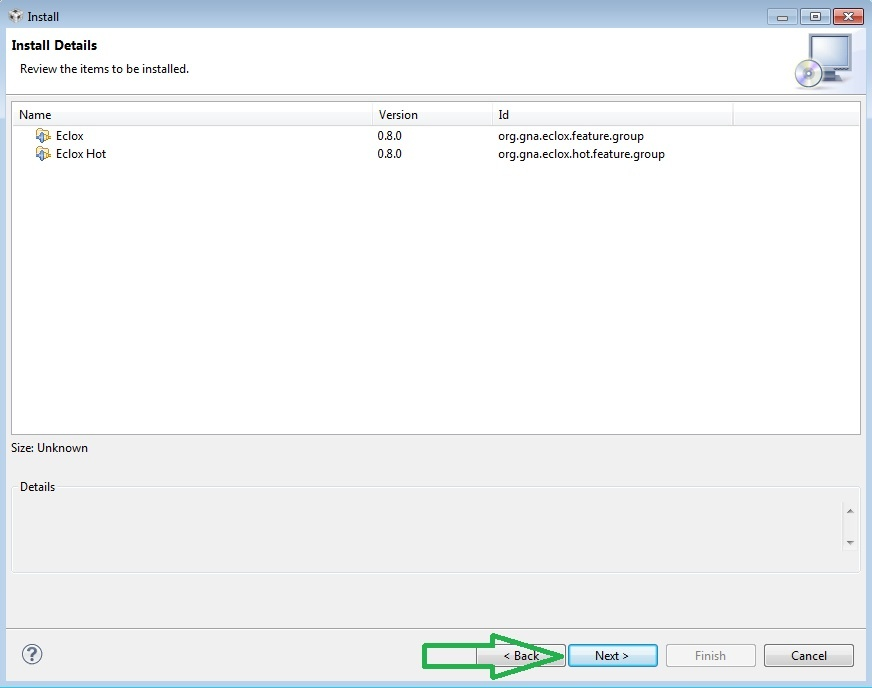The next window is a chance to review the license.You will then be presented with a security warning, as far as I am aware there are no issues with this software, but if you have any concerns then I suggest you refrain from continuing with the installation.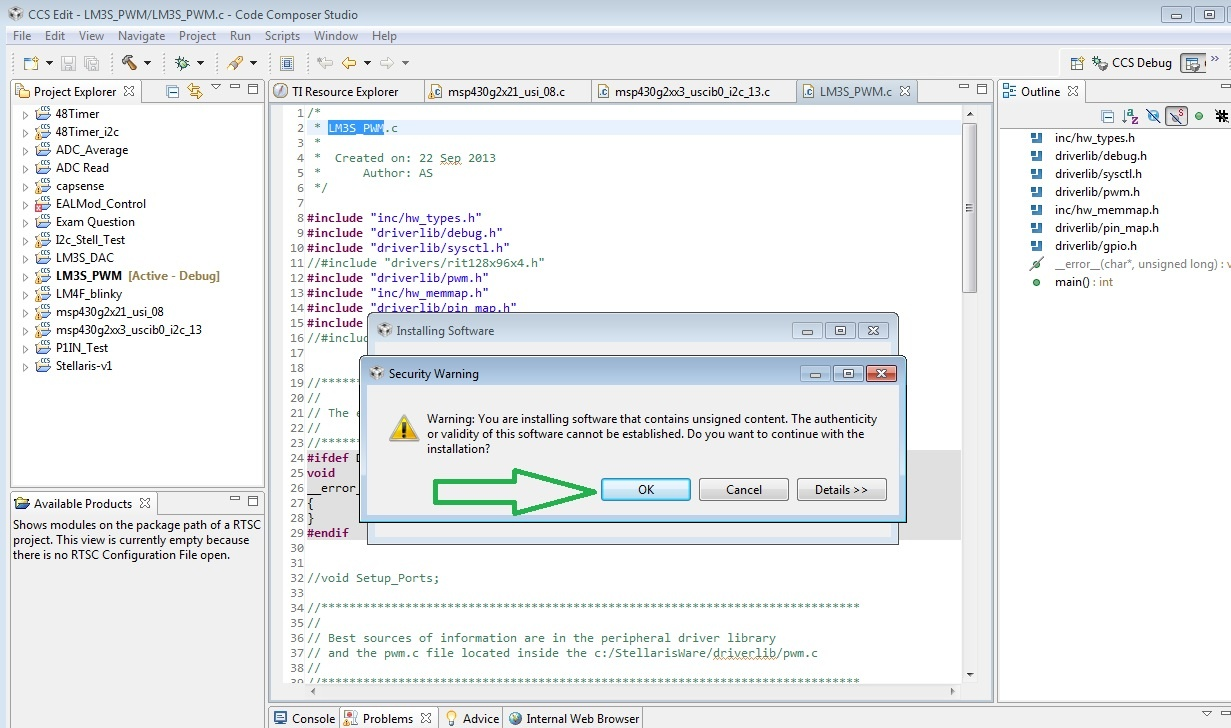All that is needed after this is to restart CCS, you should then have a new icon shown in the drop down menu, as shown in the below image.Your ready to start using Doxygen.  I intend to write some basic tutorials on the usage of Doxygen with C Programming code sometime soon when I have time.

I take great care when writing all the tutorials and articles, ensuring all the code is fully tested to avoid issues for my readers.  All this takes time and a great deal of work, so please support the site by using the Adfly links etc.  If you have found this useful or have any problems implementing, please feel free to leave a comment and I will do my best to help.